# Estimating Circuit Yields

A common exercise for all design engineers is understanding the "spreads" or yields on their circuit performance. When using SPICE, this can be done by running hundreds or thousands of iterations with tolerance distributions applied to components. From this, a graph can be produced that shows, for example, how a filter might perform when manufactured.

A typical phase of ramping a product for manufacturing is to understand how the circuit is performing relative to design predictions. At some point in the development process, you will build a single unit. And then a few units. And then a few dozen units. At each step of the way, it's important to understand how the designs compare to simulations before you flip the switch and build thousands.

Surprisingly, it doesn't take too many samples to give you an idea of how things will work out in production. Often times, the performance of a dozen or so units can accurately be extrapolated to understand the performance thousands or even millions of devices.

Side note: As we've evolved Tractor, when deciding what goes into Tractor and what goes into the QA401 application (in terms of features) we look at this in one of two ways: Is a particular feature interesting to a design engineer or a manufacturing/test engineer? Most manufacturing engineers aren't that interested in performance measurements and how they compare to simulations. Instead, they are more concerned with test pass/fail, statistical analysis of yields and throughput (among other things). Conversely, the design engineer isn't too interested in those things and instead of much more interested in how her circuit is performing relative to predictions--information provided by a detailed plot.

So, things that might be more important to a manufacturing engineer go into Tractor, and things that might be more important to a design engineer get built directly into the QA401 application. The feature being discussed in this point is likely more interesting to the design engineer, and thus has been prototyped into the frequency response plug-in.

## First, some background

Standard deviation (SD), or sigma, is an important concept when it comes to yields because it helps you understand how the circuit may perform at the extremes as you build thousands of units, assuming the yield is ultimately "normal" in its distribution. And more often than not, that's a reasonable assumption. Once you know the mean and sigma, a single sigma (+/- from the average or mean) will capture 68% of the population. Two sigmas will capture 95% of the population, and 3 sigmas will capture 99.7% of the population.

At the risk of oversimplifying a trivial case, let's say you build an inverting opamp with 10X gain using 5% resistors--a 1K and a 10K. The worst case gain could be (10K + 5%) / (1K - 5%) = 11.05 on the high side, and the worst-case gain on the low side would be 9.05. Anything between those two gains--from 9.05 to 11.05 should be expected (especially at the edges given the ways resistors are sorted). But because we can be certain the resistors won't exceed their indicated tolerance, nothing outside of that range should be expected either.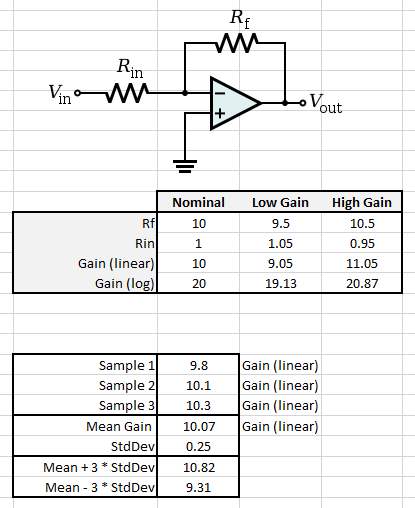Let's say you build 3 versions of this circuit and measure gains of 9.8, 10.1 and 10.3 as shown above in the spreadsheet. From this, we can compute sample statistics that we hope will map to the larger population statistics. That is, if you build 1M of the above circuit, you will be able to measure a mean and standard deviation with great accuracy. But since we only have 3 units to measure, what can we do? It's not uncommon for an engineer to think they have a population sigma from this small sample, and attempt to extrapolate as we've done above. But that would be a mistake, because if you ran the experiment again with 3 new boards, your sigma could easily be 50% higher or 50% lower. And your mean could exhibit wild shifts too.

When your confidence is low on the actual population mean and sigma, look to rely more on margin of error applied to the samples. This can help you understand where the mean might eventually end up.

## Consider the Roll of a Die

It might be helpful to think about this in terms of rolling a die. If you roll a die a thousand times, the mean (average) value of all rolls will be 3.5. If you roll it just twice, you might roll a 2 and then a 1. That would have a mean of 1.5. The 1.5 value is your sample mean, and in this case it a ways from your population mean. It's less than half! But perfectly plausible.

The the picture below, you can see the Excel formulas used to simulate rolling a die 3 times on the left. And on the right, you can see the same formulas used (not shown) for rolling a die 10 times.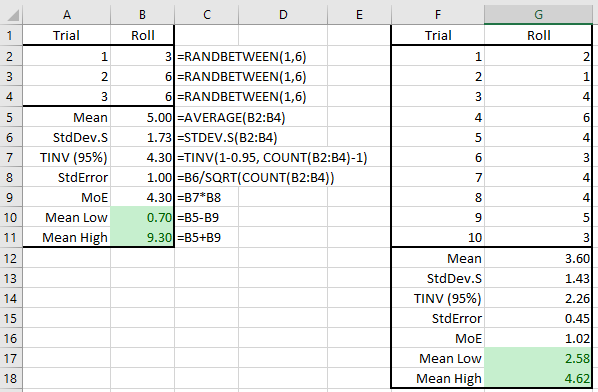We first compute the mean and standard deviation. For the standard deviation, this is using the sample (.S) version in Excel. This method uses "count-1" in the denominator, which will generally deliver a more accurate (slightly larger) estimate for smaller populations.

What is interesting in looking at the die roll data is how the MoE shrinks for the 10 rolls on the right versus the 3 rolls on the left. This is the "magic" of the math: The fewer samples you have, the more of a "barn door" your estimate becomes. And if you samples are all over the place, you get a larger window too. If you rolled a 3, 3 then 4, the margin of error would be much smaller than if you rolled a 1, 1, 6. And if you wanted a higher confidence level, say, 99.7%, then the window would get even larger still.

The final reading of the column on left (3 rolls of the die) is as follows: "Given these 3 rolls of the die, there is a 95% chance that the if we rolled the die many times the mean would be between 0.7 and 9.3." Now, given a die has value from 1 to 6, it doesn't take much math to know that with 100 percent certainty the mean will be between 1 and 6. But this is a contrived example to illustrate the concept. At higher sample counts it quickly becomes much more useful.

Note that every time you re-roll the die, your estimate changes. In the case of 3 die, it can change somewhat wildly. If you re-create the sheet in Excel, you will see that the Mean High and Mean Low capture the actual population mean of 3.5 about 19 out of 20 trials. Precisely what we expect with a 95% confidence level.

OK, so what kind of sample sizes do we need to start relying more on population statistics instead of sample margin of error? Well, it depends. You can see a graph of MoE below as a function of sample size. This is for a normal distribution with a mean of 0 and a sigma of 1.From the graph, we can see that if we have a sample size of 5 units, then we must expect our actual mean could be +/- 1.24 from our measured mean if we wanted to be 95% sure. This is large--bigger than a population standard deviation!

Notice at around 8 units, our margin of error drops to about 1 population sigma. And around 20 units, we've dropped to 0.45 sigma. So, beginning around 20 units and continuing to a few hundred units you are gently refining your estimates. But the jump from a few units to 20 units is huge at each step. In that region, you are taking a chainsaw (in a good way) to your estimates: Every unit helps a lot. 5 units is much better than 3 units. And 8 units is much better than 5 units.

In summary, as you add units to your evaluation, your MoE shrinks (converges towards the true population mean) and your sigma will likely creep up a bit.

## Back to the circuit

OK, so with the concept of Margin of Error discussed, let's go back and look at our worksheet again. And this time we'll consider the case of 95% and 99.7% confidence interval.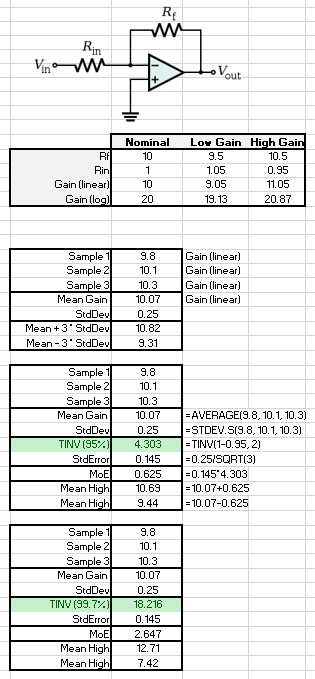In the middle section above, you can see we've opted for a confidence level that would capture around 95% of the gains we might expect. With a 95% confidence level, we'd expect our confidence interval for the gain to span from 9.44 to 10.69 based on these 3 measurements. If we wanted an even higher confidence interval of 99.7%, that would push our expected gain range even wide: from 7.42 to 12.71. But again, be careful. We've already bounded our circuit gain with the tolerance on the resistors, and the gains suggested by such a high confidence are, well, nuts. Don't torture the data!

As a quick sanity check, a small program was written to generate 1000 groups of normally distributed random numbers with mean of 0 and SD of 1. Each group was checked to see if the mean fell within the MoE. If it did, it was considered a pass. And if it didn't, it was considered a fail. The experiment ran for sample sizes of 2, 3, 4, etc, all the way up to 1000. The result was that generally, 50 out of 1000 trials failed at every sample size, indicating the math above was working as expected.The Excel math was replicated using the excellent MathNet Numerics library for the above test.

There is a very important but subtle point in the data above: A confidence level of 95% doesn't mean that 95% of the data will fall within the confidence interval. It means that 1 out of 20 times you will fail to capture the actual mean in your confidence interval. That is a very subtle but important point.

## Exposing the UI

In release 1.707, we've included code to allow you to measure several instances of a circuit's frequency response and also plot 95% confidence intervals and  mean+/-3 * sigma (see the Test Plugins->Frequency Response plugin).  We'll continue to evaluate the usability of this based on feedback, and if it continues to make sense, we'll move similar functionality to other plugins too.

What the new UI will let you measure is shown below. This particular plot reflects the performance of a group of 5 guitar overdrive guitar pedal, overlaid atop each other with statistical limits applied. The purpose of overdrive pedals is to boost the signal from the guitar and add a bit of distortion before it heads into an amp. Usually overdrive pedals will also include "tone" control, which is usually a bandpass with a peak below 1K to warm things up. Overdrive isn't fuzz. They are miles apart. Billy Gibbons, Eric Clapton and Mark Knopfler's guitar tones are rooted in overdrive. Eddie Van Halen's tone is rooted in fuzz.

This particular model of overdrive is a low-cost Kokko, commonly sold on Amazon. For this test, five units were measured with all of the knob settings at midpoint.In the plot above, we can readily compare the performance of the five different pedals. We can see the spread around 200 Hz is approaching +/- 1 dB, and that the spread really tightens up around 2K. The bandpass filter peaks around 200 Hz, and based upon roll-off rate, it appears to be a simple first order circuit, likely built with 5% parts or so.

There are a few interesting points to make on the graph. First, notice that in some places the measured data dips outside the 95% CI. This shouldn't be too surprising. As you add more samples, the MoE will tighten towards the mean--a single line and nearly all samples will reside outside of this region. Remember, the MoE lines are showing you ultimately where the mean will be, not a boundary inside which all samples must reside. The more units you run, the more the MoE should converge to a single mid-point between the +/- 3 sigma lines.

Second, note the width of the spread between the 3 sigma lines at 100 Hz. It's about 3 dB. Given the small sample size, this +/-3 sigma gap will only widen as you add samples. So keep that in mind as you evaluate performance. This looks a bit "loose" for 5 samples. That is, if you build a few hundred of these, a few customers might notice their unit sounds dramatically different than their buddy's unit.

The UI for the Frequency Response plugin now looks as follows: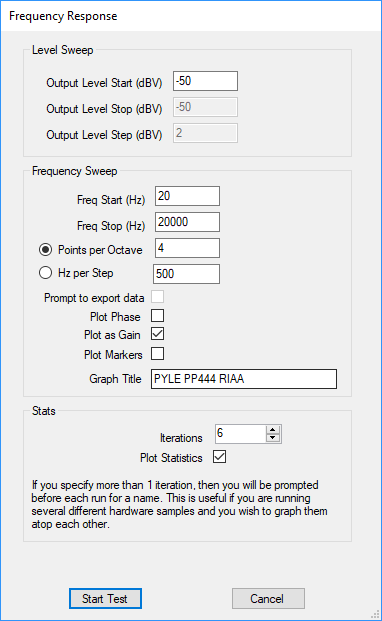The stats panel is new and it has some implications for the other UI elements. First, note the Iterations setting is key. If the Iterations box is set to '1', then the panel functions as before (with the Plot Gain and Plot Markers checkboxes being new).

You set the iteration to the number of hardware units you want to run. If you set the iteration to 2 or higher, then each run will be prefaced with a prompt asking you to enter the ID of the hardware. This could be a serial number, or board revision or a part change.

And once you've set iterations to 2 or higher, then logging is disabled and your ability to sweep at several different levels is also disabled. These might make sense to enable in the future, but the graphs generated from these statistical sweeps are pretty dense already and the aim here is to avoid readability issues.

### Update 3-Jan-2019

Below is a gain plot of 6 Pyle PP444 RIAA Phono Pre-amp. The Frequency Response plug-in to date has only shown absolute input levels. But the new checkbox noted above ("Plot as Gain") will plot gains instead of input amplitude. Note that if you are doing a sweep at different amplitudes, then you probably want the gains checkbox disabled. Otherwise, you'll get a view of gain linearity (which would be helpful if you wanted to see 1 dB compression point, for example). In the plot below, we can see the phono preamp has a gain of 50 dB at the low end, dropping to a gain of 18 dB at the high end.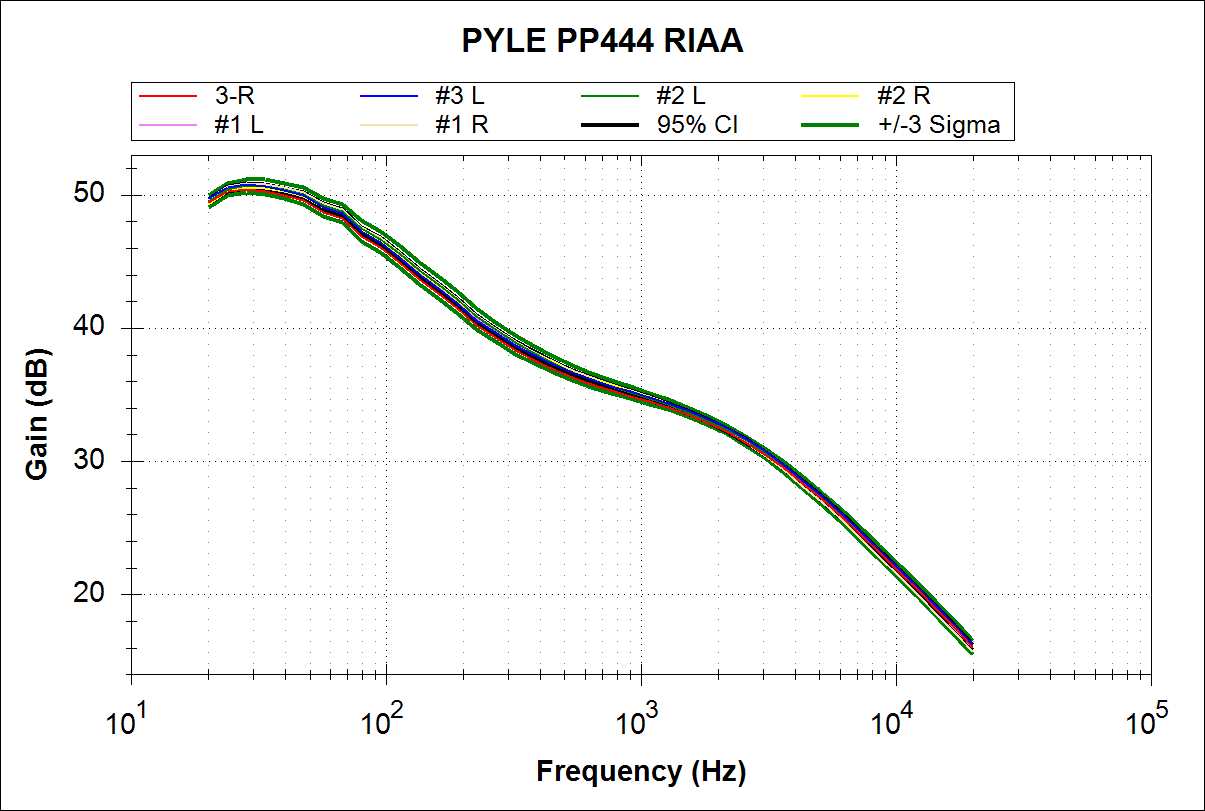This was 3 units, and left + right were measured, so that's 6 measurements total. Note the 3 sigma at the low frequency is just under 1 dB. The RIAA response is a bit wonky in the low end, but the spreads and consistency are looking tight.

In order to comment on the overall shape of the RIAA curve, we can hit the preamp with an impulse, and then apply an RIAA Record Response curve user filter, normalize to 0 dB @ 1 KHz, and we get the following:From this, we can see the preamp is about 5 dB below expected at 20 Hz, around 2 dB low at 100 Hz, and runs about half a dB high from 2K to 20K.

In a matter of a few minutes, using out-of-the-box tools included with the QA401, we're able to characterize the repeatability of a pre-amp AND visually verify the preamp's performance relative to a complex user-defined mask.

# Summary

A software change has been made in the 1.707 release to enable you to make measurements on multiple hardware devices and plot statistical information about those measurements.

Let us know if you find this useful, and we'll look to refine and drop into other plug-ins as well.